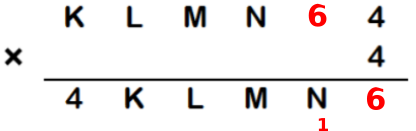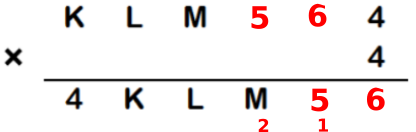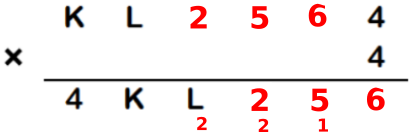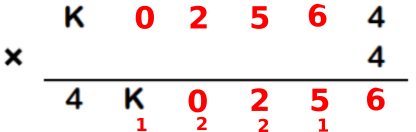You may also likeConsecutive Numbers

An investigation involving adding and subtracting sets of consecutive numbers. Lots to find out, lots to explore.Have You Got It?

Can you explain the strategy for winning this game with any target?Counting Factors

Is there an efficient way to work out how many factors a large number has?

Missing Digits

Age 11 to 14 ShortChallenge Level

Answer: K, L, M, N, P are 1, 0, 2, 5, 6.

Working it out by doing the calculation
4$\times$4 = 16, so P must be 66$\times$4 + 1 = 25, so N must be 55$\times$4 + 2 = 22, so M must be 22$\times$4 + 2 = 10, so L must be 0Now, 0$\times$4 + 1 = 1, so K must be 1.

So K, L, M, N, P are 1, 0, 2, 5, 6.

Using algebra
Suppose we are looking for the number KLMNP, and let KLMNP $=x$.
Then the top number, KLMNP4, is KLMNP0 + 4, which is $10x+4$.
The bottom number, 4KLMNP, is 400 000 + KLMNP, which is $400000+x$.

So $(10x+4)\times4=400000+x$. Solving for $x$,\begin{align}40x+16&=400000+x\\39x+16&=400000\\39x&=399984\\x&=399984\div39=10256\end{align}
So K, L, M, N, P are 1, 0, 2, 5, 6.

You can find more short problems, arranged by curriculum topic, in our short problems collection.